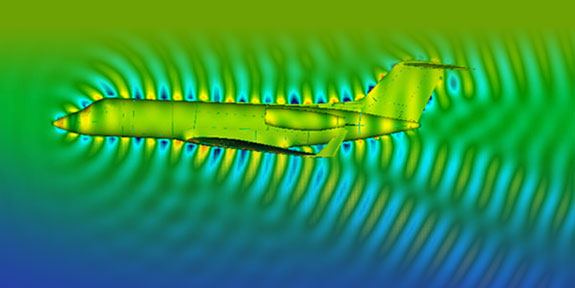# Research > Scientific Computing & Numerical Analysis## Overview

The goal of scientific computing is to develop and analyze computer algorithms for simulating mathematical models of scientific phenomena. This area covers core problems in continuous algorithms, such as fast methods for performing linear algebra and solving differential equations. At the other extreme, practitioners build software for simulating challenging physical problems, such as turbulence in fluids and crack propagation in solid materials.

CMS focuses on several aspects of scientific computing. Our Graphics & Geometry group builds accurate methods for discretizing continuous models while preserving physical invariants. Researchers in Numerical PDE work on systems with distinct behavior at different scales and the solution of equations posed on complicated geometries. Our Uncertainty Quantification group studies optimal estimation in the presence of limited information.

## Faculty

Related research groups & Centers > CD3

## Related Courses

ACM 11. Introduction to Matlab and Mathematica.
ACM 95/100abc. Introductory methods of applied mathematics.
ACM 101ab. Methods of applied mathematics.
ACM/CMS 104. Linear algebra and applied operator theory.
ACM 105. Applied real and functional analysis.
ACM 106ab. Introductory methods of computational mathematics.
CE/Ae/AM 108 ab. Computational mechanics.
ACM/CMS 113. Mathematical optimization.
ACM/CS 114. Parallel algorithms for scientific applications.
ACM/EE 116. Introduction to stochastic processes and modeling.
AM/ACM 127. Calculus of variations.
Ma/ACM 142. Ordinary and partial differential equations.
CS/CNS 171. Introduction to computer graphics laboratory.
CS/CNS 174. Computer graphics projects.
CS 176. Introduction to computer graphics research.
CS 177. Discrete differential geometry: Theory and applications.
CS 179. GPU programming.
ACM 201ab. Partial differential equations.
ACM 204. Topic: Matrix analysis.
ACM 210ab. Numerical methods for PDEs.
Ae/AM/CE/ME 214abc. Computational solid mechanics.
Ae/ACM/ME 232 ab. Computational fluid dynamics.
ACM 270. Numerical optimization.